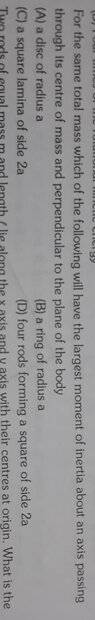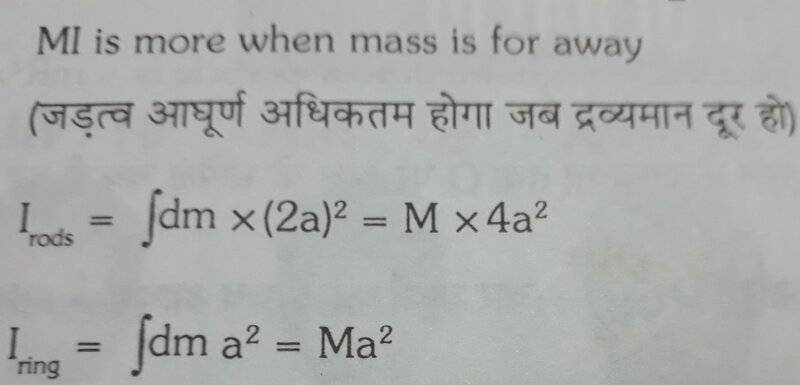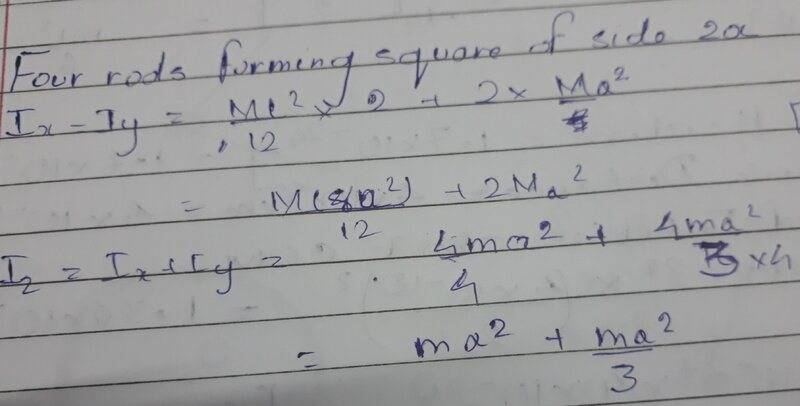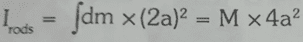# Moment of Inertia of a 4 rod system

Homework Statement:
Calculate the Moment of Inertia of each and determine the greatest:
Relevant Equations:
MOI=mr2
This was the question(The line below is probably some translation of upper line in different language)

For disc it was ma^2/2
For ring it was ma^2
For square lamina it was 2ma^2/3
For rods
It was differentThank YouTSny
Homework Helper
Gold Member
I don't know what was being calculated by this equationThe hand-written calculation is a little messy and hard to follow, but it looks correct for the 4 rods making a square.

It probably calculated the MOI of the system (because it was the solution given in the booklet)

Also my MOI came out to be
(m/4)×(8a^2)÷12 + 2×(m/4)×a^2

&&

(ma^2)+(ma^2)/3

TSny
Homework Helper
Gold Member
Also my MOI came out to be
(m/4)×(8a^2)÷12 + 2×(m/4)×a^2
Ok, you are saying that ##I_{\rm x\, axis} = I_{\rm y\, axis} = \frac{m}{4} \frac{8a^2}{12} + 2 \frac{m}{4}a^2##
That looks correct.
It would be nice to simplify the result.
&&

(ma^2)+(ma^2)/3